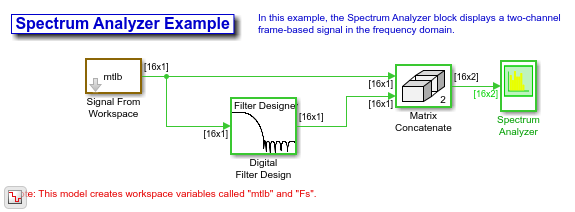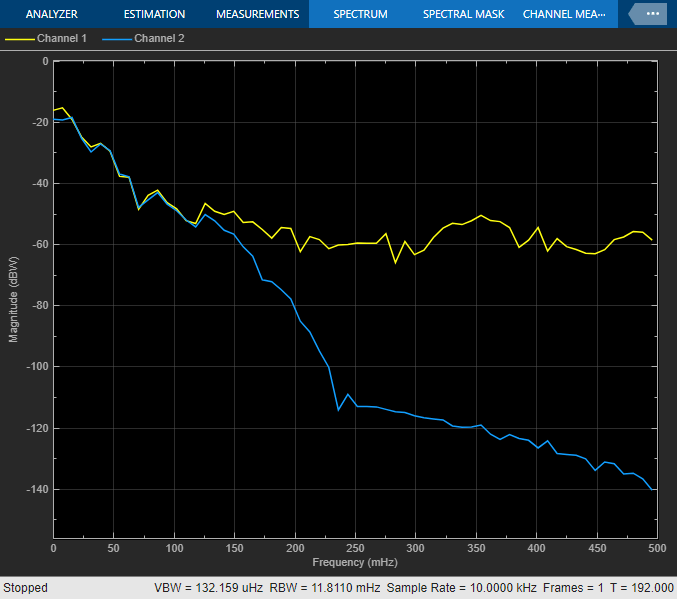# Display Frequency-Domain Data in Spectrum Analyzer

This example shows how you can use a Spectrum Analyzer block to display the frequency content of two frame-based signals simultaneously. The Spectrum Analyzer block computes the Fast Fourier Transform (FFT) of the input signal internally, transforming the signal into the frequency domain.

Open the ex_spectrumanalyzer_tut model.

model = 'ex_spectrumanalyzer_tut'; open_system(model)The Signal From Workspace block repeatedly outputs the input signal, mtlb, as a frame-based signal with a sample period of 1 second.

The Digital Filter Design block filters the input signal, using the default parameters.

open_system([model '/Digital Filter Design'])The Matrix Concatenate block combines the two signals so that each column corresponds to a different signal.

The frequency of the signals are displayed in the Spectrum Analyzer. The Spectrum Analyzer uses 128 samples from each input channel to calculate a new windowed data segment, as shown in this equation:Therefore, the FFT also has a length of 128 frequency points. Also, because Overlap (%) is set to 50, there is a buffer overlap length of 64 samples in each spectral estimate, as shown in the following equation:Run the model and view power frequency of the signals in the Spectrum Analyzer. The power spectrum of the first input signal, from column one, is the yellow line. The power spectrum of the second input signal, from column two, is the blue line.

sim(model) open_system([model '/Spectrum Analyzer'])Watch now